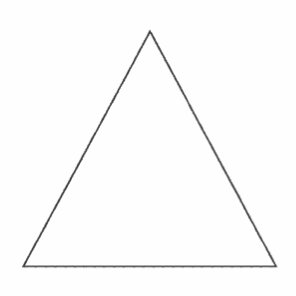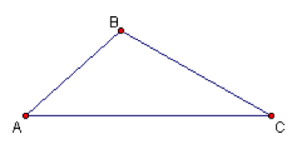### Geometric construction iii

BASIC SCIENCE JSS2 first term

Sub-Theme: Safety

WEEK 9

Geometric construction III

Performance objectives

Students should be able to:

1. Identify define and construct various triangles
2. Inscribe and circumcise circles to triangles

Content

TrianglesA triangle has three sides and three angles. It is a closed figure with three line segments as its boundary. These line segments are called sides. It has three corners called vertices. The symbol for a triangle is Δ

The three vertices are generally named by three consecutive alphabets such as A, B and C or X, Y and Z. A triangle with vertices A, B, and C is denoted by ΔABC.Tri means.... View More